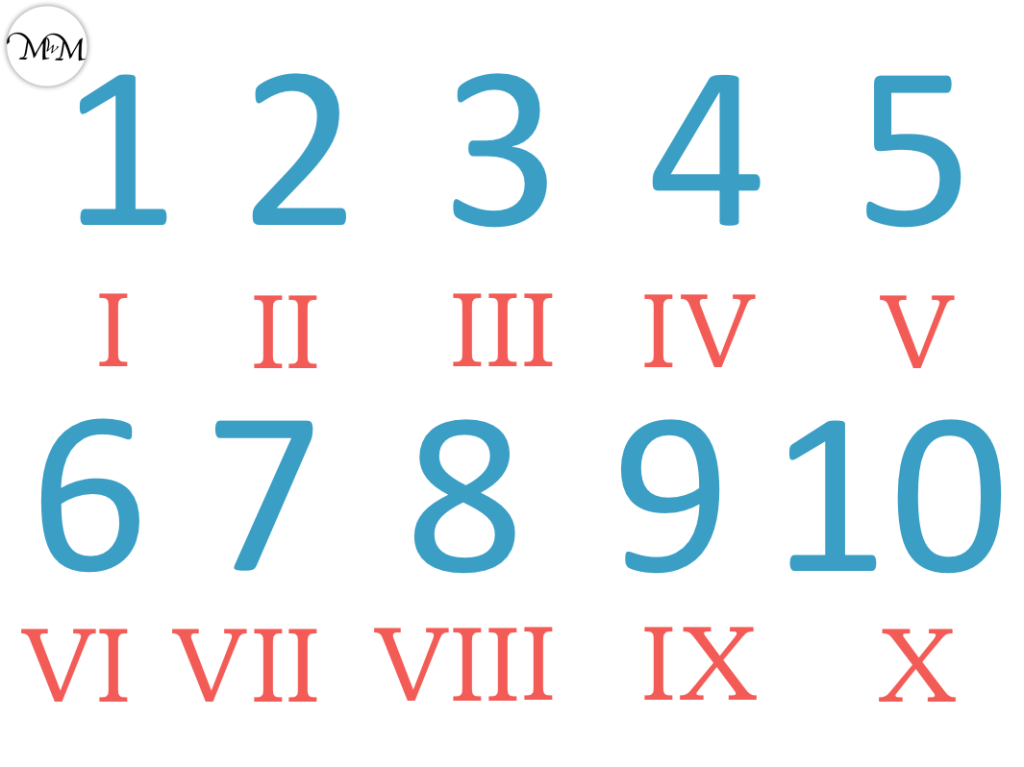• The time that each bus leaves each location is written alongside it in the same row.
• Each column shows the journey of a different bus.
• We can read the journey of each bus by reading downwards.
• The Market is the first stop and the School is the last stop.
• Later times are shown to the right.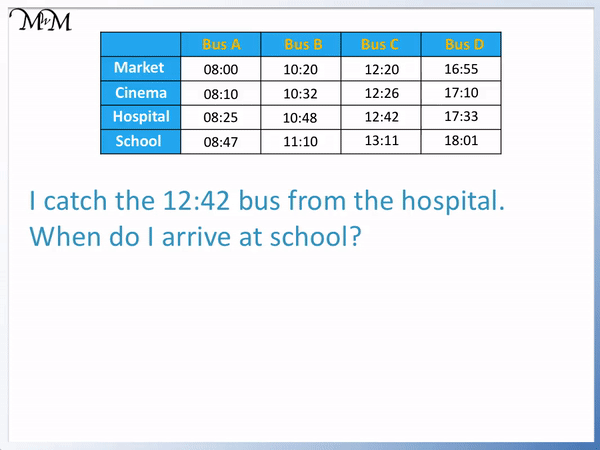• The 12:42 bus from the hospital is in the same row as the hospital
• We read across from the word hospital until we see 12:42.
• We mark this bus as the bus we will catch.
• We read downwards to see where it goes next.
• The School is the next stop and it gets there at 13:11.

Each bus travels downwards down each column of the timetable.

Each time shows when the bus arrives at the location listed in the same row to the left.• Bus B leaves the hospital at 10:48.
• Bus B gets to the school at 11:10.
• To work out the duration of the journey we find the difference between 11:10 and 10:48.
• The hours are different and so we can count from 10:48 to 11:00 and then from 11:00 to 11:10.
• There are 60 minutes in an hour and the time from 10:48 to 11:00 is 60 – 48, which is 12 minutes.
• We then have a further 10 minutes from 11:00 to 11:10.
• The journey takes a total of 22 minutes## How to Read a Bus Timetable

To read a bus timetable, look down the left column to find the name of the station you are at. The times that each bus leaves this station are in the same row to the right. Now read downwards from a chosen time to see what time this bus reaches the coming destinations.

A bus timetable is written in columns. Each new bus journey is written in its own column.

Below is an example of a bus timetable being created.

We have four different buses: Bus A, Bus B, Bus C and Bus D, which are each in their own column.We can see Bus A’s journey begins at the market at 08:00.

Its next stop is at the cinema. It leaves the cinema at 08:10.

Then the bus goes to the hospital. It leaves the hospital at 08:25.

The final stop is at the school. It arrives at the school at 08:47.

When reading a timetable, the time shown is the time that we leave that location. It is possible for the bus or train to arrive earlier than this time but if it does, it should wait at this location until the time written on the timetable.

The only case where this is not true is in the final location, where the time shown is the time that the bus or train arrives at this stop.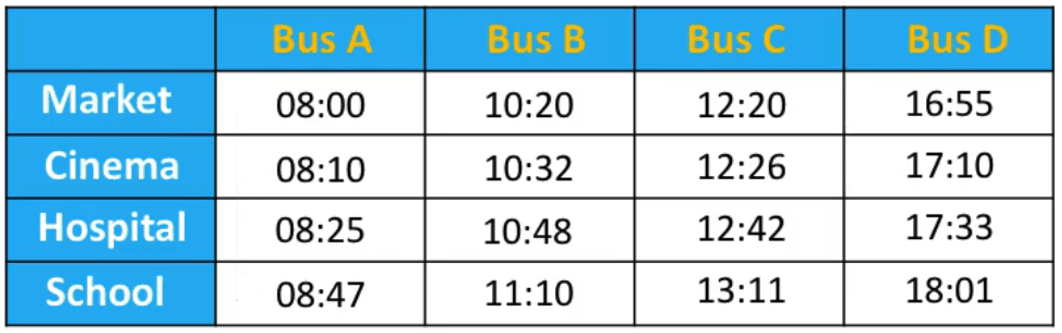We can see that Bus B leaves the market at 10:20, leaves the cinema at 10:32, leaves the hospital at 10:48 and arrives at the school at 11:10.

Bus C leaves the market at 12:20, leaves the cinema at 12:26, leaves the Hospital at 12:42 and arrives at the School at 13:11.

Bus D leaves the market at 16:55, leaves the cinema at 17:10, leaves the hospital at 17:33 and arrives at the school at 18:01.

Here is an example of reading a bus timetable.

“I catch the 12:42 bus from the hospital. When do I arrive at school?”

We first find the hospital station in the left column and read across from this row to find the bus that leaves at 12:42.We now read downwards to see the rest of the journey after it leaves the hospital. After the hospital stop, the bus arrives at the school. The bus arrives at school at 13:11.

In this next example, “I am at the cinema and it is 11:50. When is the next bus?”

We need to read the times of the different buses that leave the cinema. We read from the same row as the cinema.

The times of buses that leave the cinema are: 08:10, 10:32, 12:26 and 17:10.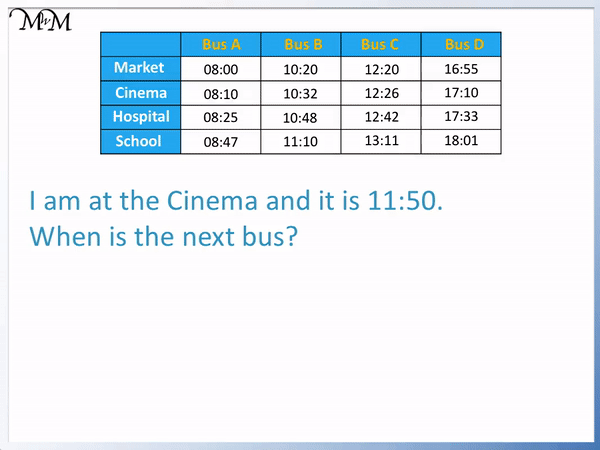It is 11:50, so I have already missed the 08:10 and 10:32 buses. The next bus is at 12:26.

In this next example we are asked, “How long does it take Bus A to get from the market to the cinema?”

We need to find the time when Bus A leaves the market and the time it arrives at the cinema. We then find the difference between these times.Reading the schedule, Bus A leaves the market at 08:00 and arrives at the cinema at 08:10.

We now find the difference between these times. The hours are the same as they are both 8 o’clock. We can see that from 08:00 to 08:10, the bus has taken 10 minutes.

In this next example we are asked, “How long does it take Bus A to get from the hospital to the school?”

Reading the bus schedule, we can see that Bus A leaves the hospital at 08:25 and arrives at the school at 08:47.Again both times have the same hour of 8 o’clock. So to find the difference between the times, we simply find the difference between the minutes.

To find the difference, we subtract.

47 – 25 = 22 and so, the journey takes 22 minutes.

In this next example we are asked, “How long does it take Bus B to get from the hospital to the school?”

Bus B leaves the hospital at 10:48 and arrives at the school at 11:10.

This time the hour times are different. One is after 10 o’clock and the next time is after 11 o’clock.Because the hours are different, we find the minutes to the next hour and count on from there.

We first find the time from 10:48 until the next hour, 11 o’clock.

There are 60 minutes in an hour so the time taken from 10:48 to the next hour is 60 – 48.

60 – 48 = 12, so the time to 11:00 is 12 minutes.

We now count on from 11:00 to 11:10. This is an extra 10 minutes.

In total, the time taken is 12 minutes plus 10 minutes.

The journey takes a total of 22 minutes.

We are now asked, “How long does it take Bus D to get from the market to the hospital?”

Bus D leaves the market at 16:55. It arrives at the hospital at 17:33.Again the hours are different and so, we count on from 16:55 to 17:00 before counting on to 17:33.

16:55 is five to 17:00 and so it is 5 minutes until 17:00.

We then have a further 33 minutes until 17:33.

In total we have 5 minutes plus 33 minutes. The journey took 38 minutes.

In these examples, we will look at examples of reading a bus timetable with buses running from Worcester to Rubery.We can see that not all stops have times written next to them. This means that the bus does not stop at this station.

If there is no time written for a station then the bus will skip this station and go directly to the next one down on the schedule with a time next to it.

In this example we have, “I catch the 07:16 bus from Fernhill. Will I be able to get off in Sidemoor?”

We first find the 07:16 bus that is in line with the Fernhill stop. We then read downwards until we find the box in line with the Sidemoor stop.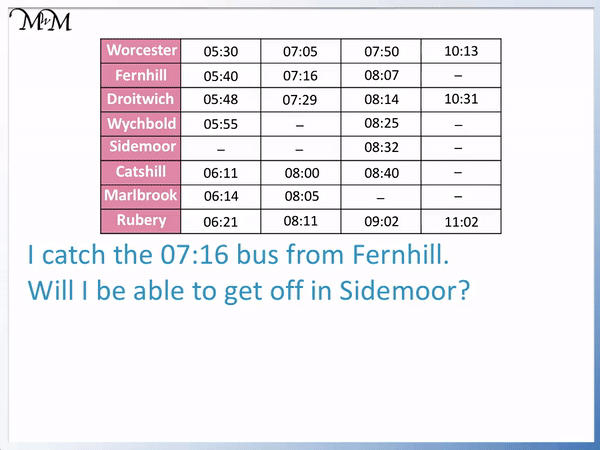We can see that after the bus leaves Fernhill at 07:16, it leaves Droitwich at 07:29. There is no time next to the Wychbold station and then there is no time next to the Sidemoor station.

This means that this bus does not stop at either of these two stations. I will not be able to get off the bus in Sidemoor.

In this next example of reading a bus timetable, “It is 06:30 and I am in Wychbold. When is the next bus?”

We can read across the row from Wychbold to see what times the bus leaves this station.A bus leaves Wychbold at 05:55, then the next bus does not arrive at Wychbold, then a bus leaves Wychbold leaves at 08:25. The final bus also does not stop at Wychbold.

If it is 06:30, then I have missed the 05:55 bus. The next bus that stops here is at 08:25.

I can catch the 08:25 bus.

In the next example, “It is 06:55 and I am in Worcester. When will I get to Catshill?”We can see that I have missed the 05:30 bus and the next bus is at 07:05.

We can read down from 07:05 to see what time this bus arrives in Catshill.

We leave Fernhill at 07:16, we don’t stop at Wychbold or Sidemoor and then leave Catshill at 08:00.

We know that we must be in Catshill at 08:00.

In this next example, “I catch the 07:50 bus from Worcester. How long will it take to get to Rubery?”

We leave Worcester at 07:50 and get to Rubery at 09:02.We can see that the hours are different so we count from 07:50 to 08:00, then from 08:00 to 09:00 and finally from 09:00 to 09:02.

There are 60 minutes in an hour so the time from 07:50 to 08:00 is 60 – 50.

This is 10 minutes.

We then have an hour from 08:00 to 09:00. This is 1 hour 10 minutes so far in total.

We then have another 2 minutes from 09:00 to 09:02. This is 1 hour 12 minutes in total.

This journey takes 1 hour 12 minutes.

## Reading Timetables with a Circular Route

Here is an example of a timetable with a loop.

We have just one bus that travels from the City to the Museum to the Beach to the Cathedral and then back to the City to complete another circuit.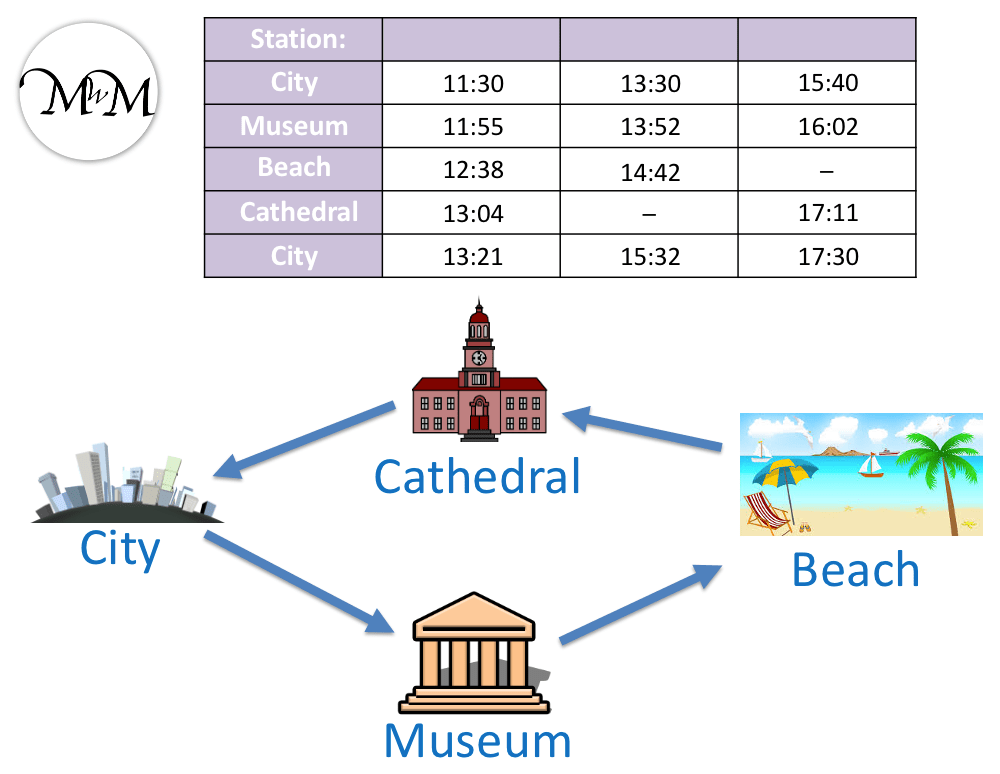The bus leaves the city at 11:30 and gets back to the city at 13:21.

The bus then waits in the city breifly.

We can see in the next column along that this bus then leaves the city again at 13:30. It gets back to the city again at 15:32.

It waits at the city again before leaving.

We can see in the next column along that this bus leaves the city at 15:40 and returns to the city at the end of the day at 17:30. It does not leave the city again.

In this example we are asked, “I am at the beach and need to get to the city. What is the latest bus I can catch?”

We first need to find at which times the bus leaves the beach.

We read the schedule and see that buses leave the beach at 12:38 and 14:42. There is no bus after this that stops at the beach. The final bus does not stop at the beach. We know this because there is no time in this row after 14:42.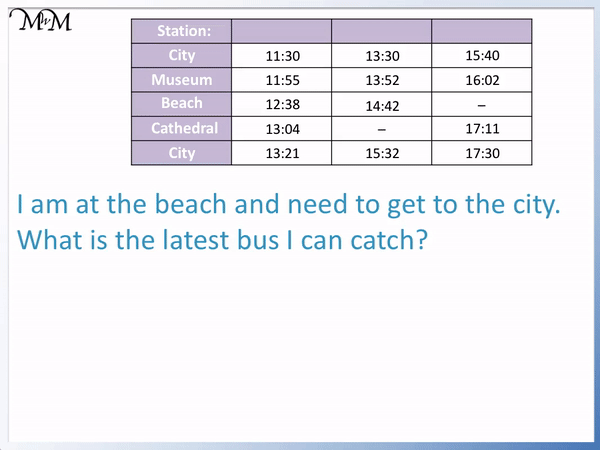In this next example we are asked, “I get on the 13:04 bus at the cathedral. What time will I get to the museum?”

We find the 13:04 bus that leave the cathedral and follow its path down.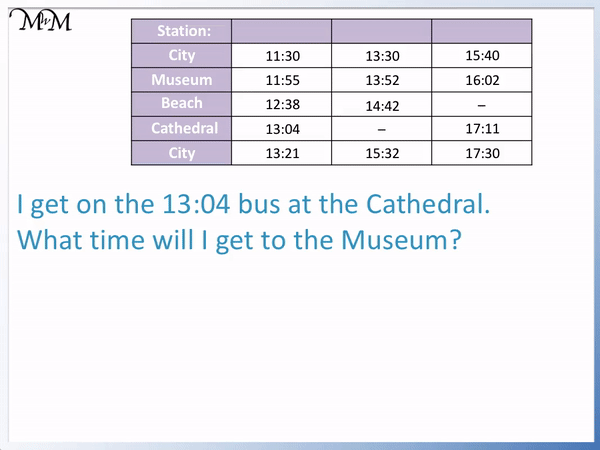After leaving the cathedral at 13:04, the bus arrives at the city at 13:21. The bus waits at the city before leaving at 13:30. We can see this at the top of the next column along.

It then gets to the museum at 13:52.

In this example we are asked, “It is 15:28 and I am in the city. How long will it take the next bus to get to the museum?”

The next bus arrives at the city at 15:32. It leaves the city at 15:40.

It gets to the museum at 16:02.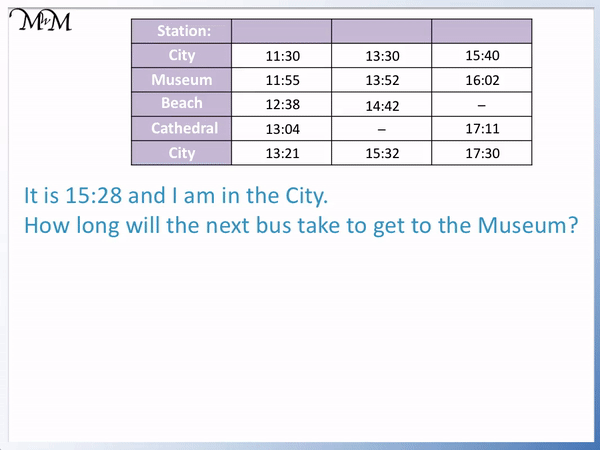The bus journey begins at 15:40 and ends at 16:02.

The hours are different and so to work out the duration, we count from 15:40 to 16:00 and then from 16:00 to 16:02.

There are 60 minutes in an hour so from 15:40 to 16:00 it is 60 – 40 minutes. This is 20 minutes so far.

We then have 2 more minutes from 16:00 to 16:02.

In total we have 22 minutes.Now try our lesson on Repeating Shape Patterns where we learn how to find missing shapes in different patterns.